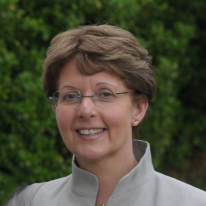# Tutorial Speakers

## Titles and Abstracts

Annie Cuyt
University of Antwerp, Belgium, and College of Mathematics and Computational Science, Shenzhen University, China.### What do Sparse interpolation, Padé approximation, Gaussian quadrature and Tensor decomposition have in common?

Abstract:

We present the problem statement of sparse interpolation and its basic mathematical and computational methods to solve it. The solution is formulated already in 1795 by de Prony and only much later expressed in terms of a generalized eigenvalue problem and structured linear system.

Input to the sparse interpolation methods are a very limited number of regularly collected samples. When considering these values as Taylor series coefficients, the problem statement easily connects to Padé approximation.

The Padé approximant denominators are closely related to formal orthogonal polynomials and Gaussian quadrature. Results on their zeroes and certain convergence properties shed new light on some computational problems in sparse interpolation.

The problem statement can also be viewed as a tensor decomposition problem, for which techniques from multilinear algebra can be used. Through the latter connection the toolkit of algorithms for sparse interpolation is further enlarged.

Matthew England
Coventry University, UK.### Real Quantifier Elimination by Cylindrical Algebraic Decomposition, and Improvements by Machine Learning

Abstract:

Given a quantified logical formula whose atoms are polynomial constraints with real valued variables, Real Quantifier Elimination (QE) means to derive a logically equivalent formula which does not involve quantifiers or the quantified variables from the original statement. For example, Real QE would reduce the statement that there exists a real solution x to the quadratic equation x^2 + bx + c = 0 to the equivalent condition on the discriminant: b^2-4c>=0.

A Cylindrical Algebraic Decomposition (CAD) decomposes n-dimension real space into a finite number of semi-algebraic cells, most traditionally in relation to a set of polynomials so that each polynomial has constant sign on each cell. A CAD for the polynomials in a formula may be used to perform Real QE, by querying a single sample point from each cell and combining cell descriptions into the quantifier free formula. CAD is the backbone of Real QE systems, used in the cases where more efficient algorithms are not applicable. CAD is implemented in a variety of computer algebra systems, as well as dedicated standalone implementations and satisfiability modulo theory solvers.

Real QE has been applied to numerous problems throughout engineering, the sciences and even economics. However, it has doubly exponential complexity which limits the scope of its use. It is thus particularly important that implementations are optimised to ensure the best possible performance. There are a variety of choices which may need to be made that can dramatically affect the runtime of such algorithms without changing the mathematical correctness of the result produces, such as the variable ordering required to compute a CAD. Such choices are in the scope of Machine Learning (ML) tools, i.e. tools that allow computers to make decisions that are not explicitly programmed, usually involving the statistical analysis of large quantities of data.

In this tutorial we will first explain the fundamentals of Real QE via CAD, before looking at the authors recent work on using machine learning to improve the performance of a CAD implementation. The latter topic may have useful lessons for those looking to apply ML to other areas of symbolic computation.

Markus Püschel
ETH Zurich, Switzerland.Abstract:

To be announced